GeeksforGeeks App
Open AppBrowser
Continue

# Areas Related to Circles – Perimeter of circular figures, Areas of sector and segment of a circle & Areas of combination of plane figures

A circle is a shape consisting of a curved line completely surrounded by an area. The circle contains points in the plane which are at the given distance from a given point, the centre, equivalently the curve traced out by a point which moves in a plane so the distance from a given point is constant. The distance between any point and the centre of the circle is called the radius (r).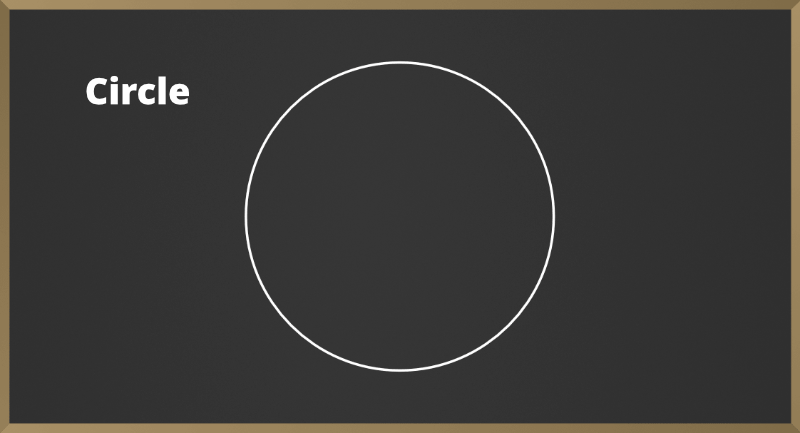### Area of a Circle

The area of the circle is computed by the formula:

πr2

• r is the radius of the circle.
• π is the ratio of the diameter of a circle to its circumference
• The value π is 22/7 (or) 3.14.

### Circumference of a Circle

The perimeter of the circle is the distance along the boundary of the circle. The perimeter is also called the circumference of a circle. Circumference will be the π  times of the diameter of the circle.

• Circumference of a Circle is 2πr.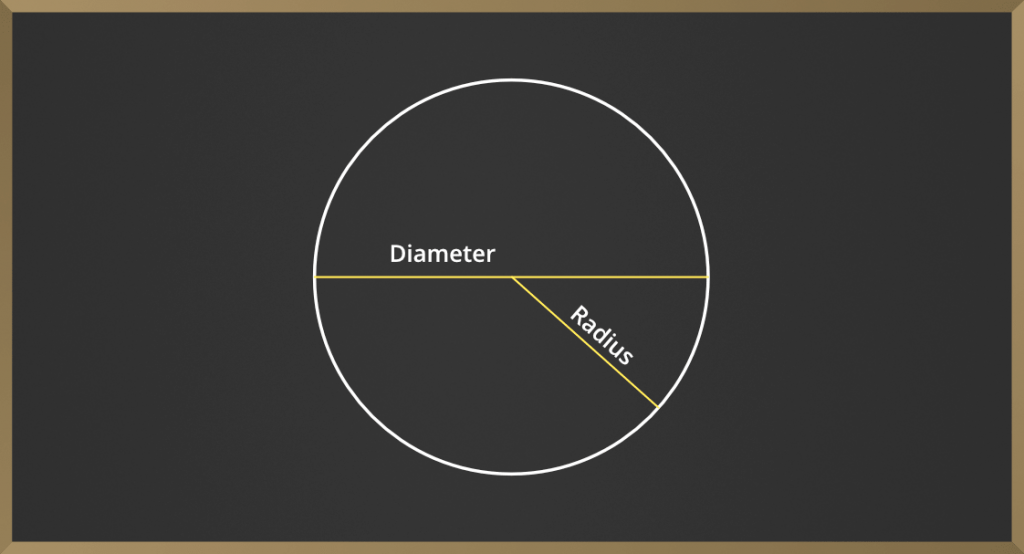### Sector

A sector of a circle is defined as the region of a circle enclosed by an arc and two radii(r). The smallest area of a circle is called the minor sector and the largest area of a circle is called the major sector.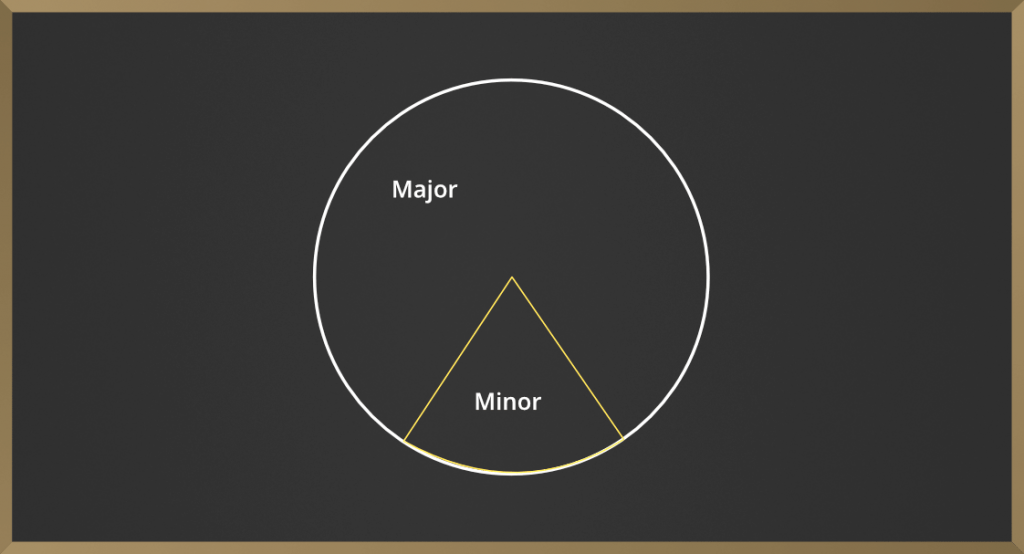### Angle of a Sector

• The angle of a sector is that angle which is enclosed within the two radii of the sector. It consists of an arc.
• A sector that has a central angle of 180° is known as a semicircle.
• A minor arc is smaller than a semicircle. A central angle that is subtended by a minor arc has a measure of less than 180°.
• A major arc is larger than a semicircle. A central angle that is subtended by a major arc has a measure larger than 180°.

### Arc

• To find the length of an arc of a circle we use the arc length formula: length = radius * θ
• An arc is a part of a curve.
• It is a portion of the circumference of the circle.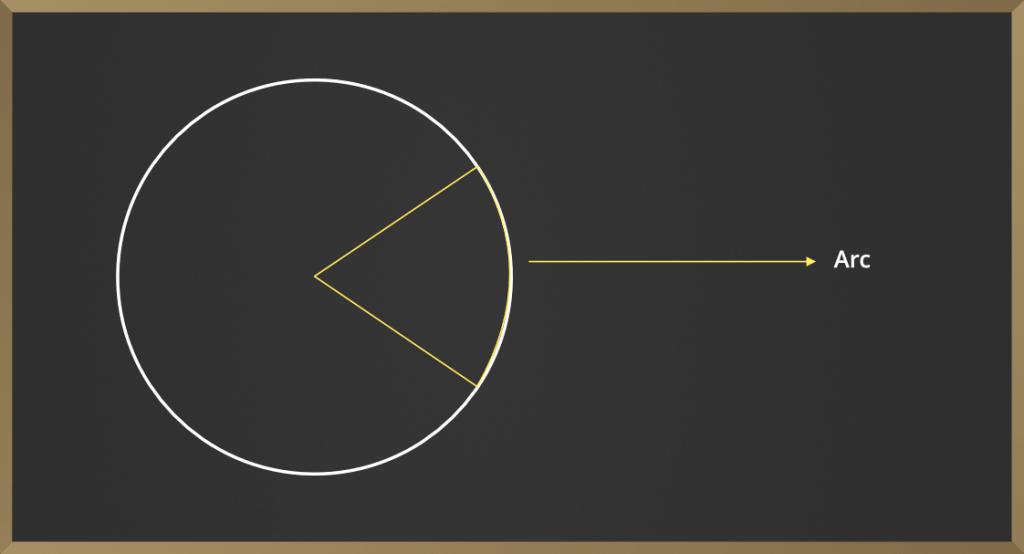### Sample Problems

Problem 1: Find the area of a sector and arc length of a circle of radius
4cm and the central angle is 2π/5.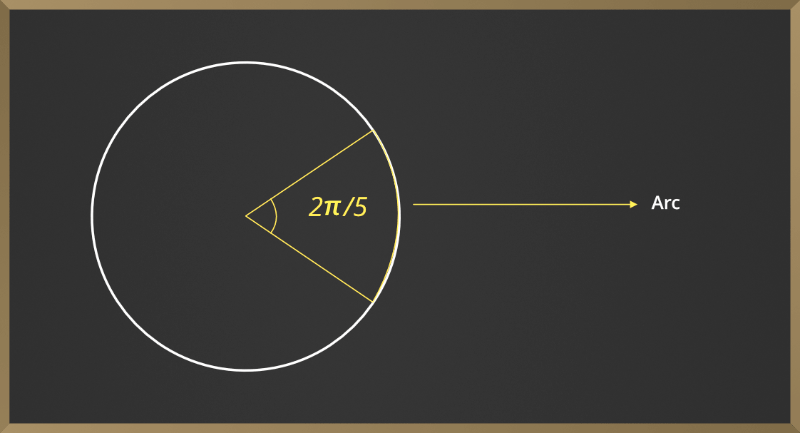Solution:

Arc length, l = 4*2π/5

= 8π//5cm.

area of a sector = 1/2θr2

= 1/2 * 2π/5 *42

= 16π/5cm2.

### Circumference of a circle when the area is given

The circumference of the circle can be referred as the linear distance around it. If the circle is opened to form a straight line then, the length of that line will be the s circumference of the circle.

To calculate the circumference of a or a given circle, we need to multiply the diameter of the circle with the π.

Problem 2: What is the perimeter of the circle whose surface area is 314.159 sq.cm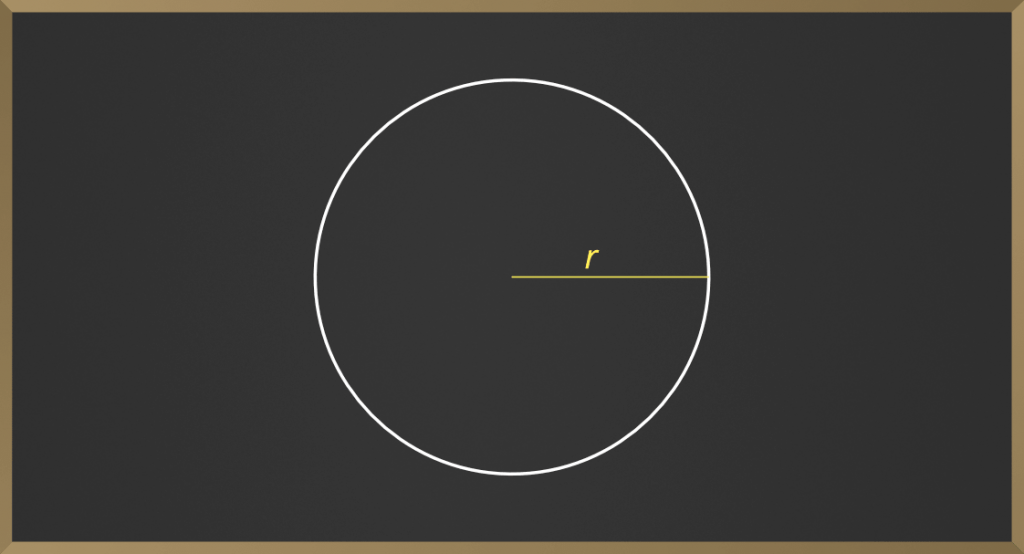Solution:

The formula of the surface area of the circle, we know:

A = π x r2

Now, substituting the value:

314.159 = π x r2

314.159 = 3.14 x r2

r2 = 314.159/3.14

r2 = 100.05

r = √100.05

r = 10 cm

C = 2 * π * r

Substitute value of r

= 2π * 10

= 2 * 3.14 * 10

= 62.8 cm.

### Partial circle area and arc length

The circle area can be also referred to as the circumference. The area of the partial circle which is also called sector can be found by the formula

[(π*r2)/360]θ

The arc length is given by

(θ/360)*(2 * π * r)

Problem 3: A circle of radius 4 units, angle of its sector is 45°. Find the area of the sector.

Solution:

Given,

Angle θ = 45°

Area of the sector

= θ/360o × πr2

= 45/360 × 22/7 × 42 = 6.28sq.units.

### Area of a Shaded Region

The shaded region is the region that can be found when one shape is inscribed within the other shape.

• The area of the shaded region can be calculated by subtracting the area of the inscribed shape area from the area of the shape which is inscribed in it.
• For example, let us assume a circle is inscribed in the triangle then the area of the shaded region is the area of the triangle minus the area of the circle inscribed in the triangle(shaded area = area of a triangle – area of a circle).

Problem 4: Find the area of the shaded region.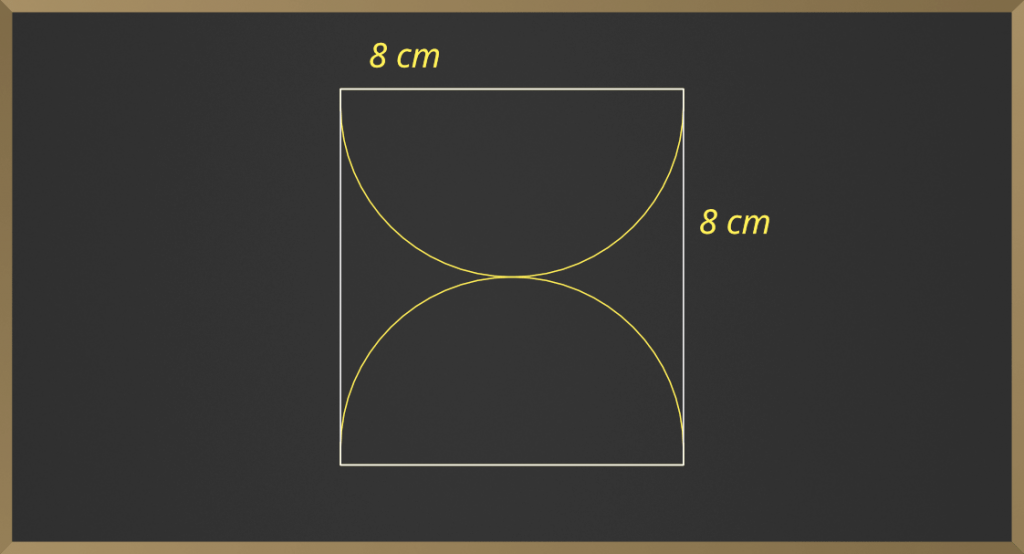Solution:

The area of shaded portion, we have to subtract area of two semicircles from the area of square

Area of shaded portion = Area of square  – (Area of semicircle + Area of another semicircle).

=  a2 – [(1/2) πr2) + ((1/2) πr2)]

= 82 – πr2

=  64 – (22/7)⋅ (7/2)2

=  64- (22/7)⋅ (7/2)⋅ (7/2)

=  64 – 38.5

=  25.5 cm2

Problem 5: Find the area of the shaded region.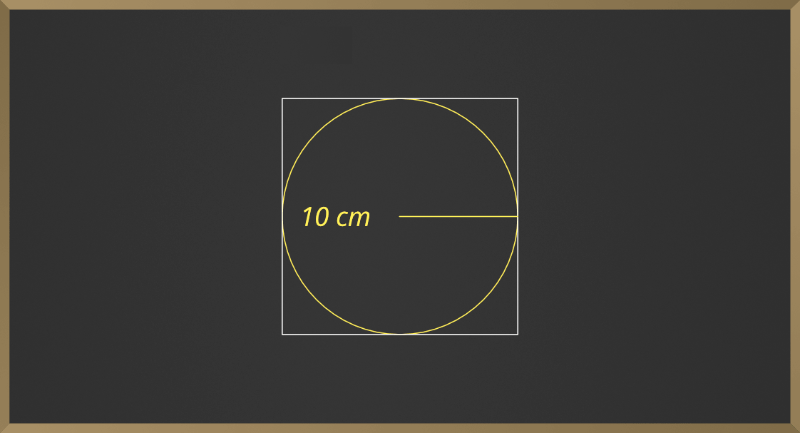Solution:

Given radius r = 10 cm

area of shaded region = area of square – area of circle inscribed inside square.

area of square= S2

diameter of the circle will be equal to length of the side of the square so

d = S = 2*10

= 20

area of square =(20)2

= 400cm2

area of circle = πr2

= 3.14 * 10 * 10

= 314 cm2

Area of shaded region = area of square – area of circle inscribed inside square

= 400 – 314

= 86cm

Therefore, area of shaded region is 86cm.

My Personal Notes arrow_drop_up
Related Tutorials